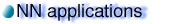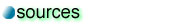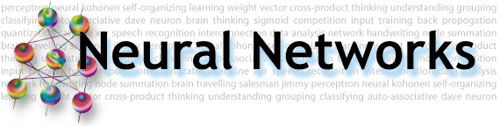Simple competitive networks:

In classification and prediction problems, we are provided with training sets with desired outputs, so backpropagation together with feed-forward networks are useful in modeling the input-output relationship. However, sometimes we have to analyze raw data of which we have no prior knowledge. The only possible way is to find out special features of the data and arrange the data in clusters so that elements that are similar to each other are grouped together. Such a process can be readily performed using simple competitive networks.

Simple competitive networks are composed of two networks: the Hemming net and the Maxnet. Each of them specializes in a different function:

 1 The Hemming net measures how much the input vector resembles the weight vector of each perceptron. 2 The maxnet finds the perceptron with the maximum value.

In order to understand how these two seemingly unrelated networks function together, we need to examine more closely the details of each one.

The Hemming net:(Fig.1) A Hemming net.

Each perceptron at the top layer of the Hemming net calculates a weighted sum of the input values. This weighted sum can be interpreted as the dot product of the input vector and the weight vector., where i and w are the input vector and the weight vector respectively.

If w and i are of unit length, then the dot product depends only on cos theta. Since the cosine function increases as the angle decreases, the dot product gets bigger when the two vectors are close to each other (the angle between them is small). Hence the weighted sum each perceptron calculates is a measure of how close its weight vector resembles the input vector.

The Maxnet:(Fig.2) A Hemming net.

The maxnet is a fully connected network with each node connecting to every other nodes, including itself. The basic idea is that the nodes compete against each other by sending out inhibiting signals to each other.

This is done by setting the weights of the connections between different nodes to be negative and applying the following algorithm:

Algorithm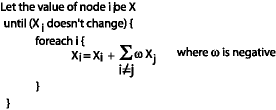Using the above algorithm, all nodes converge to 0 except for the node with the maximum initial value. In this way the maxnet finds out the node with the maximum value.

Putting them together: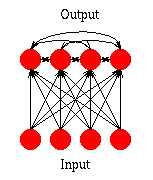(Fig.3) A Simple Competitive network.

In a simple competitive network, a Maxnet connects the top nodes of the Hemming net. Whenever an input is presented, the Hemming net finds out the “distance” of the weight vector of each node from the input vector via the dot product, while the Maxnet selects the node with the greatest dot product. In this way, the whole network selects the node with its weight vector closest to the input vector, i.e. the winner.

The network learns by moving the winning weight vector towards the input vector: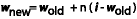while the other weight vectors remain unchanged.(Fig.4) The winner learns by moving towards the input vector.

This process is repeated for all the samples for many times. If the samples are in clusters, then every time the winning weight vector moves towards a particular sample in one of the clusters. Eventually each of the weight vectors would converge to the centroid of one cluster. At this point, the training is complete.(Fig.5) After training, the weight vectors become centroids of various clusters.

When new samples are presented to a trained net, it is compared to the weight vectors which are the centroids of each cluster. By measuring the distance from the weight vectors using the Hemming net, the sample would be correctly grouped into the cluster to which it is closest.